## Abstract

The renewed interest that is being paid by architects, project developers and local governments to integrate wind turbines with buildings is mainly required a framework to unify much data, criteria and variables to ease the design process to many architects. Therefore, this paper introduces and elaborates the systematic framework towards the efficient integration of wind technologies into new building. Moreover, it evaluates the framework effectiveness by comparing the current status of wind technologies integration into a building with the suggested status if the framework is followed.

## Keywords

Building integrated wind technology ; Integration method ; Systematic framework

## Abbreviations

IPCC , Intergovernmental Panel on Climate Change ; WTs , Wind Technologies ; BIWT , Building Integrated Wind Technology ; HAWTs , Horizontal Axis WTs ; VAWTs , Vertical Axis WTs ; WARP , Wind Amplified Rotor Platform ; IBL , Internal Boundary Layer.

## 1. Introduction

The increasing threats of climate change, along with diminishing fossil fuel energy sources, and uncertainty over the security of energy supplies, underscore the increasing value of renewable energy technologies. According to the Intergovernmental Panel on Climate Change (IPCC), buildings are responsible for one-third of global energy-related CO2 because of their dependency on fossil fuels (Urge-Vorsatz, 2007 ). As a result, it is imperative that; architects and engineers should find building׳s design ways to decrease its amount of fossil fuels consumption. One of the ways is the integration of wind technologies (WTs) into the primary building design to produce energy where it is consumed.

Building Integrated Wind Technology (BIWT) is becoming increasingly common as a green building icon to achieve energy self-sufficient building. However, the integration of WTs into buildings has not reached its goal yet. The reason is the absence of a framework that helps the architects to achieve the efficient integration. Therefore, this paper aims to introduce this efficient integration framework, which includes four stages: (1) determining site suitability; (2) determining suitable integration methods; (3) determining suitable WTs; and (4) comparing energy production with consumption. Then, this systematic framework is applied on Strata SE1 building in London, UK and the results are used to compare the building׳s current status with other integration methods when applied to the case study in its conceptual design phase. The results of these four stages and their analyses were finally combined and synthesized in the case study building to evaluate the usability and effectiveness of the suggested systematic framework.

## 2. Building integrated wind technology

Building designers are showing an increasing interest in reducing the environmental impact of their buildings. Hence, the first step is to reduce energy demands and the second is to cover most of the remaining needs of building by renewable energies. One of the useful approaches being used is BIWT (Stankovic et al., 2009 ). In this context, WTs, which have many types, can integrate into buildings in many forms. Therefore, BIWT advantages, integrated-wind technology types, methods and problems associated with integrated-wind system are illustrated in 2.1 , 2.2  ;  2.3 .

### 2.1. What are the advantages of BIWT?

Wind energy systems are omnipresent, freely available, environmental friendly, and they are considered as promising power generating sources due to their availability and topological advantages for local power generations. As a result, BIWT is becoming interesting subject to research for most of architects around the world to learn, analysis and design with. BIWT is a building that is designed and shaped with WT in mind. Therefore, a systematic framework is needed to achieve efficient BIWT. Furthermore, the trend towards BIWT is increasing because of BIWT advantages which are the following (Abohela et al ., 2011  ; Beller, 2009 ; Cace et al ., 2007  ;  Stankovic et al ., 2009 ): (a) support the WTs, (b) harness wind to be driven towards the WTs, (c) capture higher wind speeds because of the height, (d) reduce energy transmission losses, (e) reduce fossil fuel resources consumption, and finally, (f) increase CO2 savings that make a visible “green” image.

### 2.2. Integrated-wind technology: types and methods

Generally, WTs, which harness the energy from the wind by the conversion of kinetic energy into electrical one (American Wind Energy Association, 2003 ), can be divided into three main types: two types based on the axis in which the WT rotates (Horizontal Axis WTs (HAWTs) and Vertical Axis WTs (VAWTs)), in addition to the third type that includes other WTs such as Vibration and Millimeter Technologies. Moreover, each WT type has three sizes: pico (swept area fewer than 4.9 m2 ), small (swept area ranged from 4.9 to fewer than 300 m2 ) and medium (swept area equals or more than 300 m2 ).

The main methods of WTs integration into buildings vary from integration on roof to integration as an external envelope (Fig. 1 ). In addition, each main integration method has sub-methods such as integration on building side, which will be suggested for the case study building. In this context, integration on building side includes four sub-methods (Fig. 2 ): edge or corner, curved side, Aeolian Corner and Wind Amplified Rotor Platform (WARP) system.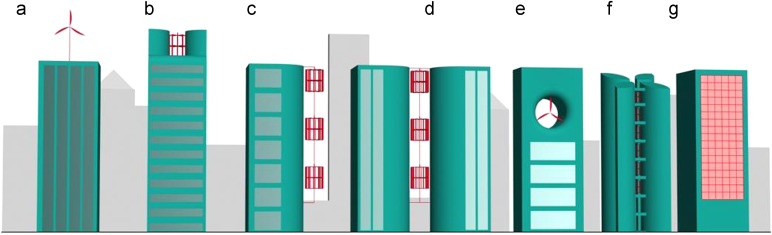Fig. 1. The main methods of WTs integration into buildings: (a) on building roof; (b) concentrator on building roof; (c) on building side; (d) between twin buildings; (e) concentrator within a building façade; (f) combined concentrator within a building façade; and (g) as an external envelop of building. Source: the authors after (Dunster, 2006  ;  Stankovic et al ., 2009 ).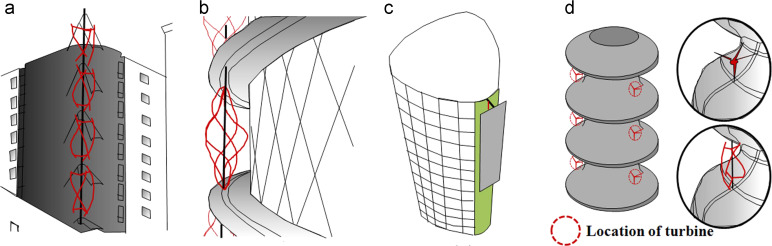Fig. 2. The sub-methods of WTs integration on building side: (a) VAWTs integrated on the curved edge of the Kinetica building in UK; (b) VAWTs integrated on the curved side of a high-rise structure; (c) the Altechnica Aeolian Tower building-augmented system; and (d) the WARP system. Source: the authors after (Dutton et al ., 2005  ; Pennsylvania State University, 2014 ; Sivakumar, 2012 ; Taylor, 2008  ;  Weisbrich and Pucher, 1996 ).

### 2.3. Problems associated with integrated-wind system

The efficient integration of WTs into buildings should overcome the following fundamental considerations. First: treating vibration from WTs by installing vibration dampening at the base and head of the WT (Breshears and Briscoe, 2009 ). In addition, acoustic treatment should be done by isolating WTs from occupants with technical or service spaces, in addition to separating between the WT and adjacent spaces. Second: designing the external envelope of building to accelerate and not disturb the wind flow towards the WT (Stankovic et al., 2009 ). Third: considering safety requirements in supporting the WTs (Beller, 2009  ;  Syngellakis et al ., 2007 ). Furthermore, maintenance requirements should be considered by a straightforward and a safe access to WTs components (Dutton et al., 2005 ). Moreover, a space within the building for WT system and a passage for cables between WT and main switchboard are required (Cace et al ., 2007  ;  Sharpe, 2010 ). Finally energy yield enlargement by the integration of multiple WTs on the same building is favorable (Cace et al., 2007 ). In a technical point of view, designing the Wind Turbine is such a complex engineering product, which requires integration of different disciplinary such as mechanical, electrical, structural as well as architectural point of view. In other words, adequate aid efforts are needed to consider the expectation of each individual aspect of design, which required multidisciplinary study of many other specialist studies. However, the presented paper presents only the architectural point of view that may encourage other specialists to add to the scientific knowledge.

## 3. The Science behind the systematic framework

Based upon our empirical work and conceptual analysis as well as related research (for example Dutton et al., 2005 ; Lysen, 1983 ; Masters, 2004 ; Mertens, 2006 ; Stankovic et al., 2009 ; Tong, 2010 ), we define four stages (Fig. 3 ) that need to be followed when designing BIWT. These four stages form a comprehensive systematic framework, which not only serves to unify and define many charts, matrices, equations and other scientific data to architects, but also serves as a platform for the efficient integration of WTs into buildings. In this context, the four stages are illustrated in the following points.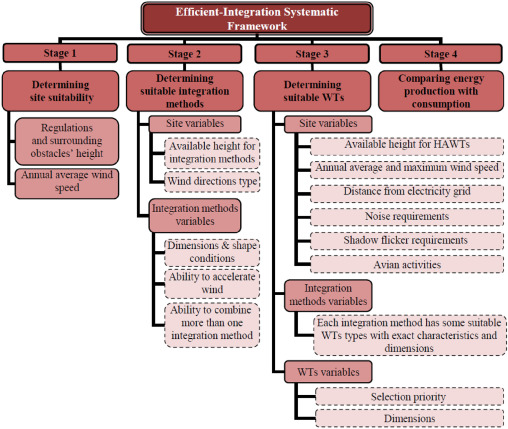Fig. 3. Systematic framework for the efficient integration of WTs into buildings.

### 3.1. Stage 1. Determining site suitability for exploiting wind technologies

According to the suggested systematic framework, our first stage is the determination of site suitability to exploit WTs. This stage is affected mainly by regulations and surrounding obstacles׳ height, in addition to annual average wind speed.

#### 3.1.1. Regulations and surrounding obstacles׳ height

Both constructions permitted height and minimum suitable height for WTs in a site should be determined and compared as they effect on site suitability for WTs. Firstly, the construction permitted height can be determined from height regulations. Secondly, the minimum suitable height for all WTs types (except HAWTs) can be determined from surrounding obstacles׳ height, because the wake regions, which are created by the surrounding obstacles, should be avoided (Mcguire, 2003  ;  Syngellakis and Traylor, 2007 ), as shown in Fig. 4 . In that sense, if constructions permitted height in the site equals or lower than the minimum suitable height for WTs, the site will not be suitable to exploit WTs.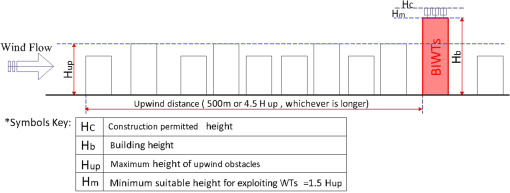Fig. 4. Building height requirements for exploiting WTs. Source: the authors after (Mcguire, 2003 ; Mertens, 2006  ;  Syngellakis and Traylor, 2007 ).

#### 3.1.2. Annual average wind speed

It has long been recognized that the annual average wind speed of the site at the minimum suitable height for WTs should be at least 4 m/s (Renewable UK, 2010 ). Therefore, firstly, the height (z ) of 4 m/s annual average wind speed (V (z )) should be estimated by the logarithmic law if the available wind speed (V (zref) ) is from the building site as following ( Lysen, 1983 ; Masters, 2004  ;  Tong, 2010 ):

 $V(z)=V(z_{ref})\times {\frac {ln\left(z-d\right)/z_{0}}{ln\left(z_{ref}-d\right)/z_{0}}}\quad \quad \quad \left(m/s\right)$
( 1)

where V (zref) is the available wind speed of the site (m/s) at reference height zref that is in the Internal Boundary Layer (IBL) and above the height d , d is the displacement height and defined as 0.75 of the average height of surrounding obstacles (m), z0 is the surface roughness length of the site (m) and can be calculated by (Masters, 2004 ):

 $z_{0}=0.5\times A_{h}\times H{'}\quad \quad \left(m/s\right)$
( 2)

where Ah is a percentage of the total area occupied by obstacles of average height Ή .

It is important to note that, if the available wind speed (VA (zA )) is from a near site, the height (z ) is determined as ( Masters, 2004 ):

 $V(z)=V_{A}(z_{A}){\frac {ln\quad {\frac {\left(z-d\right)}{z_{0}}}}{ln\quad {\frac {\left({\delta }_{I}-d\right)}{z_{0}}}}}\times {\frac {ln\quad {\frac {{\delta }_{I}}{z_{0A}}}}{ln\quad {\frac {z_{A}}{z_{0A}}}}}\quad \quad \left(m/s\right)$
( 3)

where VA(zA) is the available wind speed (m/s) at a height zA , z0A is the surface roughness length of the site (m) where the VA(zA) is measured and can be calculated by Eq. (2) , δI is the IBL height at a distance x from the site to the edge of the IBL (m) and can be calculated as follows ( Lysen, 1983  ;  Masters, 2004 ):

 ${\delta }_{I}=0.28z_{0}{\left[{\frac {x}{z_{0}}}\right]}^{0.8}\quad \quad \left(m\right)$
( 4)

Then, if constructions permitted height in the site equals or lower than the determined height (z ), the site will not be suitable to exploit WTs.

### 3.2. Stage 2. Determining suitable integration methods

The second stage identifies the suitable integration methods or that are sorted by priority. In this regard, the selection is affected by both site and integration method variables.

#### 3.2.1. Site variables

Site variables that effect on the selection of suitable integration methods are classified in the following divisions:

• Available height for integration methods : Integration on roof and in a concentrator on roof methods can only be used above building height, i.e. in the distance between building height and construction permitted height. In addition, other methods can only be used under building height, i.e. in the distance between the minimum suitable height for WTs and building height. Furthermore, each integration method has height conditions. Therefore, by comparing building height with minimum suitable height for WTs and constructions permitted height, some integration methods cannot be used.
• Wind directions type : It can be uniform or weakly unidirectional or strongly unidirectional or bi-directional. Therefore, each integration method has suitable and non-suitable wind directions׳ types. Hence, for any exact site, there are some non-suitable integration methods that should be excluded. For instance, if the site has uniform wind direction type, as in the case study building׳s site, some integration methods should be excluded such as the integration between twin buildings and in concentrator within a building façade, in addition to all sub-methods of the integration on building side except the WARP system.

#### 3.2.2. Integration methods variables

Integration methods׳ variables that effect on the selection and arrange the priorities of suitable integration methods are grouped in the following categories:

• Dimensions and shape conditions : Any building has designing conditions such as the inability to separate into two towers, the attachment to another building, the inability to shape near a circular plan …etc.., which lead to the exclusion of unsuitable methods.
• Ability to accelerate wind : It is compared to a free standing WT at the same location. Further, it is varied for different integration methods. Therefore, the priority of integration methods׳ selection or order should belong to integration methods with the highest acceleration value. For example, the WARP system has acceleration value around 1.80 V ( Dutton et al ., 2005  ;  Weisbrich and Pucher, 1996 ), which is higher than the value of the integration in a combined concentrator within a building façade that vary according to the sub-method from 0.78 V to 1.44 V ( Hughes and Chaudhry, 2011  ;  Mertens, 2006 ). As a result, the priority of selection or order between the two methods belongs to the WARP system.
• Ability to combine : The ability to combine more than one integration method together through the same building is suitable for some integration method and not for others. For instance, the using of the WARP integration method leads to the exclusion of all integration methods except the integration on roof, in a concentrator on roof and as an external envelope of building.

### 3.3. Stage 3. Determining suitable wind technologies

Once the suitable integration methods are determined in stage two, the suitable WT for each integration method can be suggested according to the site, integration method and WTs variables. In that sense, stage three determines the suitable WT׳s type, characteristics, size with specified dimensions and numbers. It is important to note that the average values of several WT׳s characteristics, which effect on its selection, are concluded by studying the WT׳s products that can be integrated with buildings and are produced by reliable manufacturers.

#### 3.3.1. Site variables: understand the built-environment wind resource

Site variables that effect on the selection of suitable WT׳s type and characteristics are grouped in the following categories:

• Available height for HAWTs : The minimum suitable height for HAWTs can be determined by avoiding the disturbed regions, which are created by the surrounding obstacles. Therefore, the minimum suitable height for HAWTs should be higher than two-times the surrounding obstacles׳ height, particularly that within one km upwind; and 500 m downwind for the prevailing or exploited wind directions ( Mcguire, 2003  ;  Syngellakis and Traylor, 2007 ). In this context, by comparing the minimum suitable height for HAWTs with building dimensions and constructions permitted height, some integration methods cannot exploit HAWTs. For example, if constructions permitted height in the site equals or lowers than the minimum suitable height for HAWTs; all integration methods cannot exploit HAWTs.
• Annual average and maximum wind speed of the site: The WT cut-in speed, where WT starts to generate usable power, should be lower than the annual average wind speed at the integration method׳s position. In addition, the WT cut-out speed, where WT shuts down immediately to avoid damaging, should be higher than this annual average wind speed. Furthermore, the WT survival speed, where WT withstands without damage, should be higher than the maximum wind speed at the integration method׳s position ( Stankovic et al ., 2009  ;  Tong, 2010 ).
• Distance from electricity grid : If the building site is not remote, i.e. not away more than approximately 400 m from the electricity grid (Noaman, 2012 ), off-grid WT such as savonius with medium size should be excluded. Hence, in remote sites, DAWT with medium size (on-grid WT) should be excluded.
• Noise requirements : The Environmental Protection Law no. 4 of 1994 and its executive regulations determined the legal limit for noise level at different urban types. Moreover, the selected WTs shouldn’t cause overall noise more 5 dB (A) than these legal limits for the "worst case" i.e., during the night at a wind speed of approximately 8 m/s ( Al-Shemmeri, 2010  ;  Minister of State for Environmental Affairs, 1994 ). For instance, if the urban type is considered residential areas on a main road, the selected WTs should have sound pressure level (Lp,n ) lower than 53.4 dB(A), which is estimated by the formula:
 $Overallsound\quad pressure\quad level=10\quad log({10}^{0.1L_{P,n}}+$${10}^{0.1backgroundnoise})\quad \quad \quad \left(dB\left(A\right)\right)$
( 5)

where overall sound pressure level is the legal limit for sound pressure level of background noise, which equals 50 dB (A) for the shown example, plus 5 dB (A), i.e. equals 55 dB (A) (American Wind Energy Association, 2009 ; Al-Shemmeri, 2010  ;  Minister of State for Environmental Affairs, 1994 ). In this case, two blades HAWT, three blades HAWT (with medium size) and Co-Axial multi rotor (with pico size) should be excluded.

• Shadow flicker requirements: Shadow flicker, which happens when the sun passes behind the WT blades as they rotate, tends to be more noticeable in buildings with windows oriented to the WTs and away by less than 300 m from the WT ( Giovanello and Kaplan, 2008  ;  Stankovic et al ., 2009 ). The impact area of shadow flicker can be determined from the sun path chart of the country (Fig. 5 ). Therefore, if there are buildings with windows oriented to the WT at the impact area, WTs types that cause shadow flicker should be excluded. These types could be as the followings: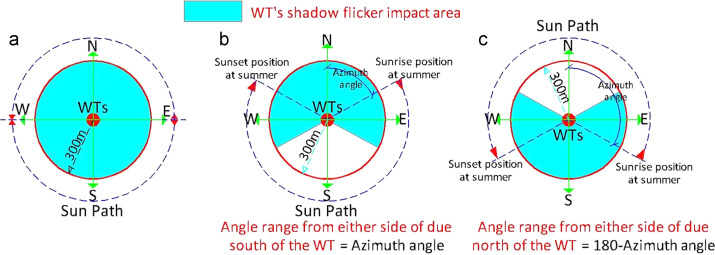Fig. 5. WT׳s shadow flicker impact area at sun path chart of countries: (a) near the equator; (b) north of the equator; (c) south of the equator. Source: the authors after (Giovanello and Kaplan, 2008  ;  Stankovic et al ., 2009 ).
• Co-axial multi rotor
• H-rotor (with pico and small sizes)
• Darrieus Helical twisted blades (with small size)
• Darrieus with blades in the form of Savonius scoops (with small size)
• Darrieus with Savonius blades on the central mast (with small size).
• Avian activities in surrounding sites : In sites that have avian activities (i.e. 120 m away from hedgerows or water courses or any wildlife habitat (Gadawski and Lynch, 2011 )), WTs types that do not provide avian protection should be excluded in all integration methods except integration in a concentrator within a building façade and in a concentrator on building roof (excluding Aeolian Roof and Between two shrouds sub-methods), because these methods provide avian protection. In this regard, WTs types that have a threat to avian are the followings:
• Dual-Rotor HAWT
• Co-Axial multi rotor

#### 3.3.2. Integration methods variables: characteristics and dimensions

After excluding the unsuitable WTs by the site variables, each integration method could exclude the other unsuitable WTs types, because each method has requirements for WTs characteristics and dimensions, as shown in Table 1 .

Table 1. Design parameters for suitable WTs at two integration methods, which will be suggested for the case study building as examples, in addition to the excluded WTs types by the design parameters. Source: the authors after (Dutton et al ., 2005  ; USA Humdinger Wind Energy LLC., 2015 ; USA Humdinger Wind Energy LLC., 2015  ;  Weisbrich and Pucher, 1996 ).
Integration methods Design parameters for suitable WTsa Excluded WTs׳ typesa
Characteristics Dimensions
Integration method Maintenance Yaw mechanism
WARP system S L/ Mo N
• Rotor diameter or height≤60% (Hb – Hm)
• Rotor diameter or height or width ≤22.8% of D2 .
• VAWT: Savonius (M)
• Other WT : Millimeter WT/Hybrid WT
As an external envelope of building E
• WT׳s swept area for millimeter WT equals 0.0005 m2 and for Vibration technology equals 0.03 m2
• HAWT
• VAWT
• Other WT : Bladeless WT/Hybrid WT

a. Symbols Key : M (with medium size), S (On building side), E (As an external envelope of building), Mo (Moderate), L (Little), N (No), Hb (building height), Hm (minimum suitable height for WTs) and D2 (the building dimension which faces the prevailing wind flow).

#### 3.3.3. Wind technologies variables

Once the suitable WTs types, characteristics and dimensions are determined by the variables of both site and integration methods, WTs variables are used to choose an exact WT type and number. In this context, WTs variables are the followings:

• Selection priority: It can depend on the WT power coefficient, cost, product׳s availability or designer׳s choice. In addition, there is an opportunity to combine among priorities, such as depending on the WT product׳s availability with taking into account the power coefficient and the cost.
• WT׳s dimensions : They effect on the WTs number at each integration method. In addition, both building and integration method dimensions conditions effect on this number. For example, if the suggested integration method is on building side (WARP system), number of WTs can be calculated and expressed as follows:

 $WTs\quad number\quad at\quad WARP\quad system=2\times \left[{\frac {Building\quad {\mbox{height}}-{\mbox{Suitable}}\quad height\quad for\quad WT}{1.66\times {\mbox{WT}}\quad height}}\right]$
( 6)

Note that: the fraction of calculating WTs number between brackets in Eq. (6) should be approximated to the lowest integral number, by the authors after Dutton et al. (2005) .

### 3.4. Stage 4. Comparing energy production with consumption

After identifying the site suitability in stage one and suitable both integration methods and WTs in stages two and three, the total annual energy consumption of the building and the annual energy output of suitable WTs at suitable integration methods should be calculated and compared. Firstly, the total annual energy consumption of the building can be calculated by multiplying the area of each building use by average energy use per unit floor area. Secondly, the annual energy output of the WTs (Eturb ) can be expected mathematically as (Masters, 2004 ):

 $E_{turb}=P_{turb}\times 8760\times average\quad wind\quad speed\quad probability\quad per\quad year\times average\quad wind\quad speed\quad probability\quad at\quad exploited\quad wind\quad directions\quad \quad (Watts.\quad h/yr)$
( 7)

where average wind speed probability at exploited wind directions is the percentage of average wind speed frequency hours at exploited wind directions and can be calculated by using the wind rose diagram of the site over a year (see the wind rose in Table 2 ); average wind speed probability per year is the percentage of average wind speed frequency hours per year and can be calculated from Eq. (8) ; and Pturb is the power output of a WT at average wind speed (Watts) and can be calculated from Eq. (9) ( Lysen, 1983  ;  Tong, 2010 )

 $The\quad percentage\quad of\quad avarage\quad wind\quad speed\quad (i.e.\quad from\quad V_{in}\quad to\quad V_{out})\quad frequency\quad hours\quad per\quad year=$$exp\left[-{\frac {\pi \quad {V_{in}}^{2}}{4\quad {V_{avg}}^{2}}}\right]-$$exp\left[-{\frac {\pi \quad {V_{out}}^{2}}{4\quad {V_{avg}}^{2}}}\right]$
( 8)

where Vin is the cut-in speed (m/s), Vout is the cut-out speed (m/s), and Vavg is the annual average wind speed of the site at WT height (m/s) that can be calculated from Eqs. (1) or (3)

 $P_{turb}=1/2\quad \rho AV^{3}C_{p}\quad {\eta }_{t}\quad \quad \left(Watts\right)$
( 9)

where ηt is the electrical converting efficiency and can be calculated by multiplying generator efficiency (ηgen ), gearbox efficiency (ηgear ≈0.95) if used and electric efficiency (ηele ≈0.8) (Al-Shemmeri, 2010 ); Cp is the power coefficient of the WT; V3 is a cubic function of the wind speed at WT height in the site (m3 /s3 ); ρ is the air density at the WT installation height (kg/m3 ) and can be calculated from Eq. (10) ; and A is the cross-sectional area through which the wind passes (m2 ) and can be calculated from Eq. (11) (Tong, 2010 )

 $\rho =P/R\quad T\quad \quad (kg/m^{3})$
( 10)

where P is the local air pressure (Pa) at WT installation height, T is the local air temperature (°K) at WT installation height and R is the gas constant (287 J/kg K).

 $A\quad (for\quad HAWT)=\pi \quad r^{2}\quad Or\quad \quad A\quad (for\quad VAWT)=$$D\times H\quad \quad \quad \left(m^{2}\right)$
( 11)

where r is the radius of the HAWT-rotor (m), π is a mathematical constant that equals 3.14, D is the rotor diameter (m) and H is the rotor height (m).

Table 2. Case study comparisons and lessons learned from current and proposed status according to the first stage of the systematic framework.
Stage 1: Site suitability
Current status Proposed status
• The building did not exploit all suitable height as it only exploited the height from 127.5 m till 147.9 m (BFLS, 2014 ).
• The building site can exploit WTs from height 105 m till 147.9 m, because:
• The construction permitted height (147.9 m ) is much more than the minimum suitable height for WTs which equals 105 m (as upwind buildings׳ height=70 m).
• The height where the annual average wind speed equals 4 m/s is 93 m . It is calculated from Eq. (3) where V(z) =4 m/s; VA(zA) =4 m/s in Brixton, London at zA =16 m; d =52.5 m; z0 =5 m; z0A =0.5 m; and δI =279.96 m (calculated from Eq. (4) where x =3760 m from site to upwind edge of the IBL) (WindFinder.com GmbH & Co. KG., 2015 ).

Finally, the comparison between the annual energy output of the WTs and the annual energy consumption of the building is performed by any of the following methods:

• Determine the share (%) of the building energy consumption to be provided by the WTs (this percentage should be equals to, or lower than, the total annual energy output of WTs).
• Select, from among suitable integration methods, the methods to be used.
• Decide on the use of all suitable integration methods.

## 4. Case study building: examining the systematic framework

This part includes analytical case study of international buildings integrated with WTs to evaluate the effectiveness of the systematic framework. The study chooses Bahrain World Trade Center in Bahrain; Strata SE1 in UK; Shanghai Tower in China; and City House in UK. The selected buildings are analyzed by comparing the current status of WTs integration in these buildings and the suggested status if the systematic framework is followed. This section applies the proposed framework on Strata SE1 building. Moreover, the analysis results of the other three buildings are introduced.

The Strata SE1 (Fig. 6 ), which is constructed in June 2010 by BFLS Architects, is the first high-rise building (147.9 m) in the world with cladding-enclosed WTs. At the same time, a comprehensive sustainability strategy from project concept to post-occupation has been developed and implemented in the building. This residential building consists of 36,610 m2 total floor area which consumes 625,000 kWh/yr when fully operational (Bogle Flanagan Lawrence Silver (BFLS), 2014 ; Council on Tall Buildings and Urban Habitat (CTBUH), 2014  ;  Stankovic et al ., 2009 ).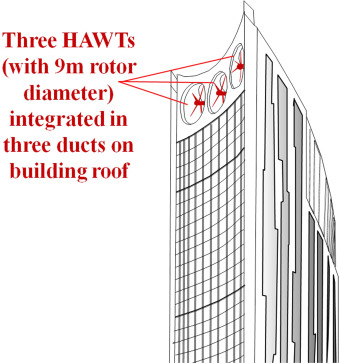Fig. 6. Perspective view of the WTs at strata building. Source: The authors after Bogle Flanagan Lawrence Silver (BFLS), 2014 ; Council on Tall Buildings and Urban Habitat (CTBUH), 2014  ;  Stankovic et al ., 2009 .

Based upon the suggested systematic framework, the first stage is to determine the site suitability to exploit WTs. This stage is applied on Strata SE1 site and compared the result to the current status, as shown in Table 2 .

Then, the second stage of the suggested systematic framework identifies the suitable integration methods or that are sorted by priority. In this regard, this stage is applied on Strata SE1 building and compared to the current integration methods, as shown in Table 3 and Fig. 7 .

Table 3. Case study comparisons and lessons learned from current and proposed status according to the second stage of the systematic framework.
Stage 2: Integration methods
Current status Proposed status
• Integration in a duct (three circular tubes) on building roof.
• It exploits an angle range up to ±45° from the south west wind direction (Stankovic et al., 2009 ).
• It changes the wind speed by 0.9 V (compared to a free standing WT at the same location) because of the tube design (Stankovic et al., 2009 ).
• More suitable integration methods could be:
• Integration on building side (WARP system) which exploits all wind directions and accelerates the wind speed by 1.80 V (Fig. 2 (d)) (Dutton et al ., 2005  ;  Weisbrich and Pucher, 1996 ).
• Integration as an external envelope of building which exploits all wind directions and changes the wind speed by less than natural wind speeds at the same height due to friction with the building envelope (Fig. 1 (g)).
• These integration methods are selected according to the following variables:
• Site variables: the building height equals the construction permitted height and much higher (by 22.5 m) than the minimum suitable height for WTs. In addition, wind directions type in the site is uniform, because 58% (i.e. less than 60%) of the wind come from one wind direction (SSW) plus an angle range up to ±75°, as shown in Fig. 7 .
• Integration methods variables: firstly, building design can be shaped near a circular plan. Secondly, the priority of selection among the rest integration methods belongs to the methods with the highest acceleration value (i.e. WARP system). Finally, WARP system integration method excludes the using of combined concentrator within the building method.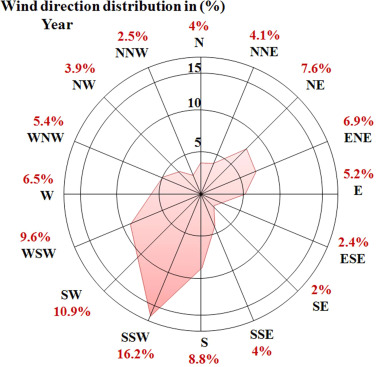Fig. 7. The wind rose diagram of Brixton, London. Source: the authors after WindFinder.com GmbH & Co. KG. (2015) .

Once the suitable integration methods are determined for the Strata SE1 building in stage two, the suitable WT for each selected integration method can be suggested by the third stage. In that sense, stage three is applied on Strata SE1building and compared to the current integrated-WTs, as shown in Table 4 .

Table 4. Case study comparisons and lessons learned from current and proposed status according to the third stage of the systematic framework.
Stage 3: Integrated-wind technologies
Current status Proposed status
• The building integrates with three HAWTs that have the following characteristics: Five blades HAWT (Norwin 19 kW that manufactured by NORWIN A/S, Denmark); rotor diameter=9 m; cut-out speed=16 m/s; sound pressure level≤63.4 dB(A); avian risks; fixed yaw; gearbox; high maintenance requirements; maximum Cp ~0.4;and ηgen ~0.9 ( Bennetsen, 2012  ;  Bogle, 2011 ).
• The used WTs have some inappropriate characteristics such as its number and dimensions which did not exploit all available suitable height.
• More suitable WTs number, types and characteristics for integration on building side (WARP system) could be Six VAWT and with the following characteristics: Savonius (small size); 7.98 m rotor width and height; cut-in speed <9.2 m/s; cut-out speed >9.2 m/s; survival speed >30.2 m/s; on-grid WTs if the grid allowed; total sound pressure level <53.4 dB(A); not cause shadow flicker; without yaw mechanism; not cause avian risks; little or moderate maintenance requirements; Cp ~0.54; and moderate cost.
• These integrated-WTs are selected according to the following variables:
• Site variables:
• HAWTs can be used from only 140 m (as upwind buildings׳ height is 70 m and downwind buildings׳ height is 45 m) till 147.9 m.
• The annual average wind speed of the site at the integration method≈9.2 m/s (calculated from Eq. (3) where wind acceleration=1.80 V; VA(zA) =4 m/s in Brixton, London at zA =16 m; and z =126.5 m (average height between minimum suitable height for WTs and building height); d =52.5 m; z0 =5 m; z0A =0.5 m; and δI ≈279.96 m). Besides, the maximum wind speed≈30.2 m/s, which is calculated from Eq. (3) where wind acceleration= 1.80 V; VA(zA) =13 m/s in Brixton, London at zA =16 m; and z =126.5 m (WindFinder.com GmbH & Co. KG., 2015 ). Hence, any WTs type that have cut-in speed >9.2 m/s; cut-out speed <9.2 m/s; survival speed <30.2 m/s should be excluded.
• The site is not remote, so off-grid WT should be excluded.
• The site is located in an urban type that is considered residential areas on a main road. Therefore, WTs types that have sound pressure level higher than 53.4 dB(A) should be excluded.
• The site is located in a distance less than 120 m from hedgerows, thus WTs types that have a threat to avian should be excluded.
• Around the site at the shadow flicker impact area, there are buildings with windows oriented to the WT (Fig. 5 (b)); consequently WTs types that cause shadow flicker should be excluded.
• Integration methods variables (the case of integration on building side by the WARP system in Table 1 ):
• WTs characteristics : WTs have little or moderate maintenance and without a yaw mechanism.
• WTs dimensions : Rotor diameter, height or width should not exceed 7.98 m (as building width that faces the prevailing wind direction equals 35 m). Further, rotor height of VAWT should not exceed 25.7 m. In addition, rotor diameter of HAWT should not exceed 4.7 m.
• WTs types : HAWT (Multi blades (pico size)) with 4.7 m rotor diameter or VAWT (Savonius (small size), Magnetic levitation (small size) or Cycloturbine (small size)) with 7.98 m rotor width and height.
• WT variables : Selection priority is depended on the designer who chooses VAWT (Savonius (small size)) for integration by WARP method and excludes suitable WTs for integration as an external envelope of building because they are unavailable products. After that, WTs number in WARP method can be calculated from Eq. (6) where building height=147.9; suitable height for WT=105 m; and WT height=7.98 m.

After identifying the site suitability in stage one and suitable both integration methods and WTs in stages two and three, the total annual energy consumption of the building and the annual energy output of both current and suggested WTs are calculated and compared, as shown in Table 5 .

 The annual energy output of current integrated WTs≈10,440 kwh/yr , which is calculated from Eq. (7) where: Pturb ≈2696.2 W, which is calculated from Eq. (9) where: ρ =1.212 kg/m3 (calculated from Eq. (10) where p =98865.2 Pa and T =284.3 °K at 147.9 m (WindFinder.com GmbH & Co. KG., 2015 )) Total A =190.9 m2 (calculated from Eq. (11) where r =4.5 m for the current WT) Vavg ≈4.4 m/s calculated from Eq. (1) where wind acceleration=0.9 V; V(zref) =4 m/s at zref =93 m; d =52.5 m; z0 =5 m; and z =147.9 m Cp =0.4 for the current WTs ηt =0.684 (calculated by multiplying ηele =0.8, ηgen =0.9 and ηgear =0.95 of the current WTs) Vavg probability per year≈0.85 (calculated from Eq. (8) where cut-in speed=2 m/s and cut-out speed=16 m/s for the current WTs) Vavg probability at exploited wind directions (±45° from the SW wind direction)=0.52 (calculated from the wind rose diagram of Brixton, London in Fig. 7 ). The annual energy consumption=625,000 kWh/yr when fully operational (BFLS, 2014 ). Based on the previous calculations, only 1.7% of annual energy consumption can be provided by the current integrated-WTs . Stage 4: Comparison between energy production and consumption Current status Proposed status The proposed integrated-WTs can produce 612,768 kWh/yr , which is calculated from Eq. (7) where: Pturb ≈74896.959 W, which is calculated from Eq. (9) where: ρ =1.214 kg/m3 (calculated from Eq. (10) where p =99,122 Pa and T =284.4 °K at 126.5 m (WindFinder.com GmbH & Co. KG., 2015 )) Total A =382.1 m2 (calculated from Eq. (11) where D and H =7.98 m for each suggested WT) Vavg of the site at the integration method≈9.2 m/s (previously calculated in Table 4 ) Cp =0.54 for the suggested WTs ηt =0.768 (calculated by multiplying ηele =0.8 and ηgen =0.96 of the suggested WTs). Vavg probability per year≈0.934 (calculated from Eq. (8) where cut-in speed=2 m/s and cut-out speed=25 m/s for the suggested WTs) Vavg probability at exploited wind directions (all directions)=1 (from the wind rose diagram of Brixton, London in Fig. 7 ). The annual energy consumption=625,000 kWh/yr when fully operational (BFLS, 2014 ). Based on the previous calculations, a 98% of annual energy consumption can be provided by the proposed integrated-WTs .

The detailed analysis on Strata building given above is also applied on Bahrain World Trade Center in Bahrain; Shanghai Tower in China; and City House in UK to determine the effectiveness of suggested framework. Based on the detailed analysis on Strata building and the comparative analysis on the other three buildings, the results in Table 6 and Fig. 8 can be concluded. It׳s important to clarify that these analytical and comparative results obtained by applying different stages of proposed systematic framework concerned only better BIWT designs and that results do not consider other functional or aesthetic aspects which may current status of case study buildings achieved better.

Table 6. The results of comparison between current status and proposed integration of the case study buildings.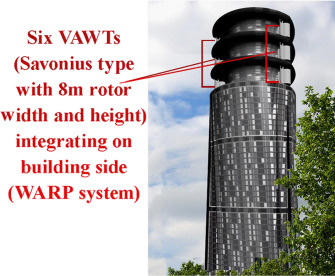Fig. 8. Perspective view of the selected integration method of WTs when applying the suggested framework on Strata SE1 building.

From the results of comparison between current status and proposed integration of the case study buildings, the suggested framework can be effective in determining the site suitability for exploiting WTs, the suitable integration methods and WTs. Therefore, this framework can be a useful tool for architects to design BIWTs.

## 5. Conclusions

There is a rising demand for renewable energy technologies due to escalating targets for CO2 reduction and increasing fuel costs. In addition, wind integrated technologies have expanded rapidly, and whilst well specified, well sited turbines could make very useful contributions in urban environments. Generally, many WTs types can integrate with new buildings by varied methods. Besides, there is no preferable WT or integration method in general. However, each integration method into specific building and site has the most preferable WT that makes the determination of suitable integration method and WT more complex.

In this regard, this research offers a systematic framework of four stages for unifying and defining all wind variables and technologies that based on scientific first principles and step logic that led to a unifying framework for architects. This systematic framework can help architects to achieve the best BIWT designs through the determination of: (a) site suitability for exploiting WTs; (b) suitable integration method; (c) suitable WT׳s type, number, dimensions and characteristics for each selected integration method; and finally, (d) annual energy production and its share of building demand. From the case study analysis, it can be concluded that, the suggested framework can be successful in achieving the efficient integration of WTs into buildings, as it made a positive move in the energy consumption share of Strata building as well as the other three cases. This efficient integration can be achieved, providing that this process is part of the building early design phase. Furthermore, the general concluded guidelines to design BIWT for higher energy production are:

• As a rule, the construction permitted height in the building site should achieve the required conditions for site suitability.
• Increasing either building height or building dimension which faces the prevailing wind flow means increasing the wind exposed area that can be exploited for WTs integration. It is important to note that increasing the building height leads to higher energy production from the WTs than increasing the building dimension, which faces the prevailing wind flow. Thus, increasing the building height is considered the best solution to exploit the increase of both wind speed with height and wind exposed façade that can be exploited for WTs integration.
• It is preferable to avoid, as much as possible, the increase of building dimension which is in the same direction with the prevailing wind flow because it increases the building energy demand while not providing more wind exposed area for WTs integration.
• In brief, WTs can integrate with any building type and shape because of the wide range of WTs and integration methods where the suitable ones for building can be found and selected.

For presenting the results from the proposed framework, the site and building data in addition to the preference methods are required as follows:

• Site data: wind data, wind directions׳ distribution, prevailing vertical wind direction on site, obstacles height at exploited wind directions within the determined upwind and downwind distance, urban type, distance from electricity grid, avian activities in surrounding site, the position of city according to the equator and existing of building in the shadow flicker impact area.
• Building data: building dimensions (height, width and depth), construction permitted height, building shape conditions and either the total annual energy consumption or the area of each use in the building.
• Preference methods: determining a method to choose from suitable WTs and to compare energy production from the WTs with energy consumption in the building.

A systematic framework for efficient integration of WTs into a building has been proposed, and its effectiveness has been assessed. Afterwards, this framework can aid architects in order to gather scientific background related to BIWTs and ease architects׳ dealing with many charts, matrices, equations and other scientific data.

In a technical point of view, designing a comprehensive systematic framework is such a complex task which requires integration of different disciplinary such as mechanical, electrical, structural as well as architectural point of view. However, the presented paper presents only the architectural point of view that may encourage other specialists to add to the scientific knowledge. With further progress, one should anticipate the evolution of more scientific perspective. The intended spirit of this perspective was not limited, but encourages new and different thinking that is steeped towards a comprehensive systematic framework.

## Acknowledgments

The authors would like to thank the reviewers for their insightful comments for the improvement of the manuscript.

### Document informationPublished on 12/05/17
Submitted on 12/05/17

Licence: Other

### Document Score0

Views 36
Recommendations 0